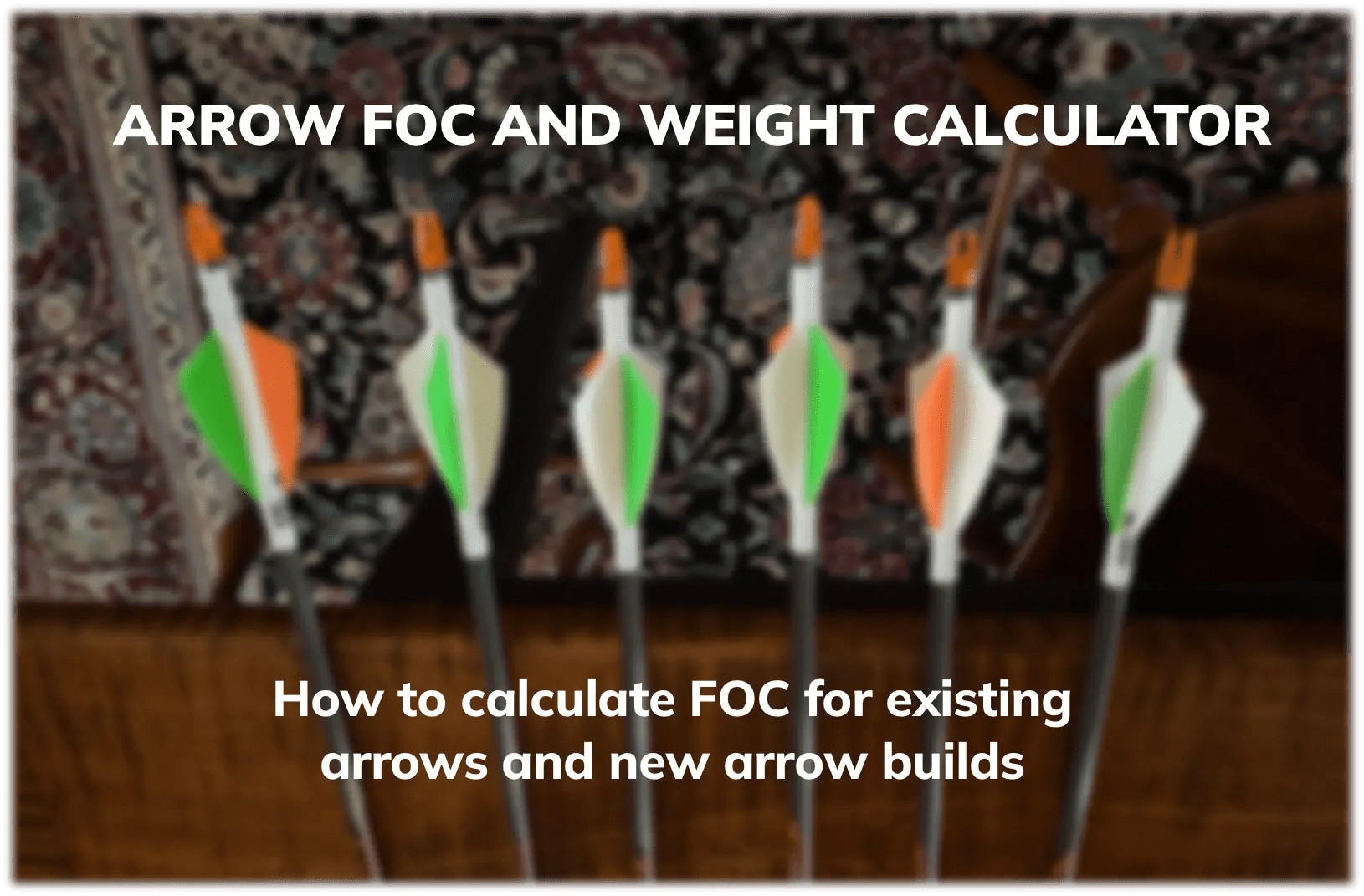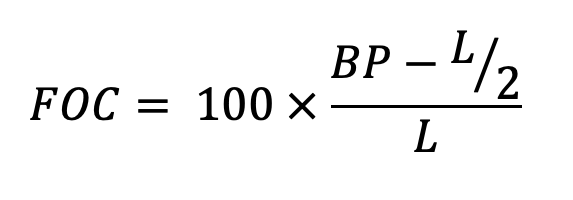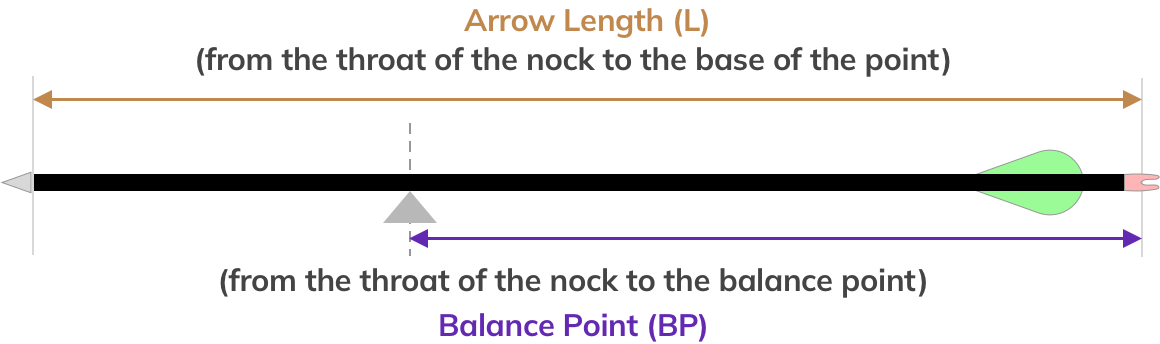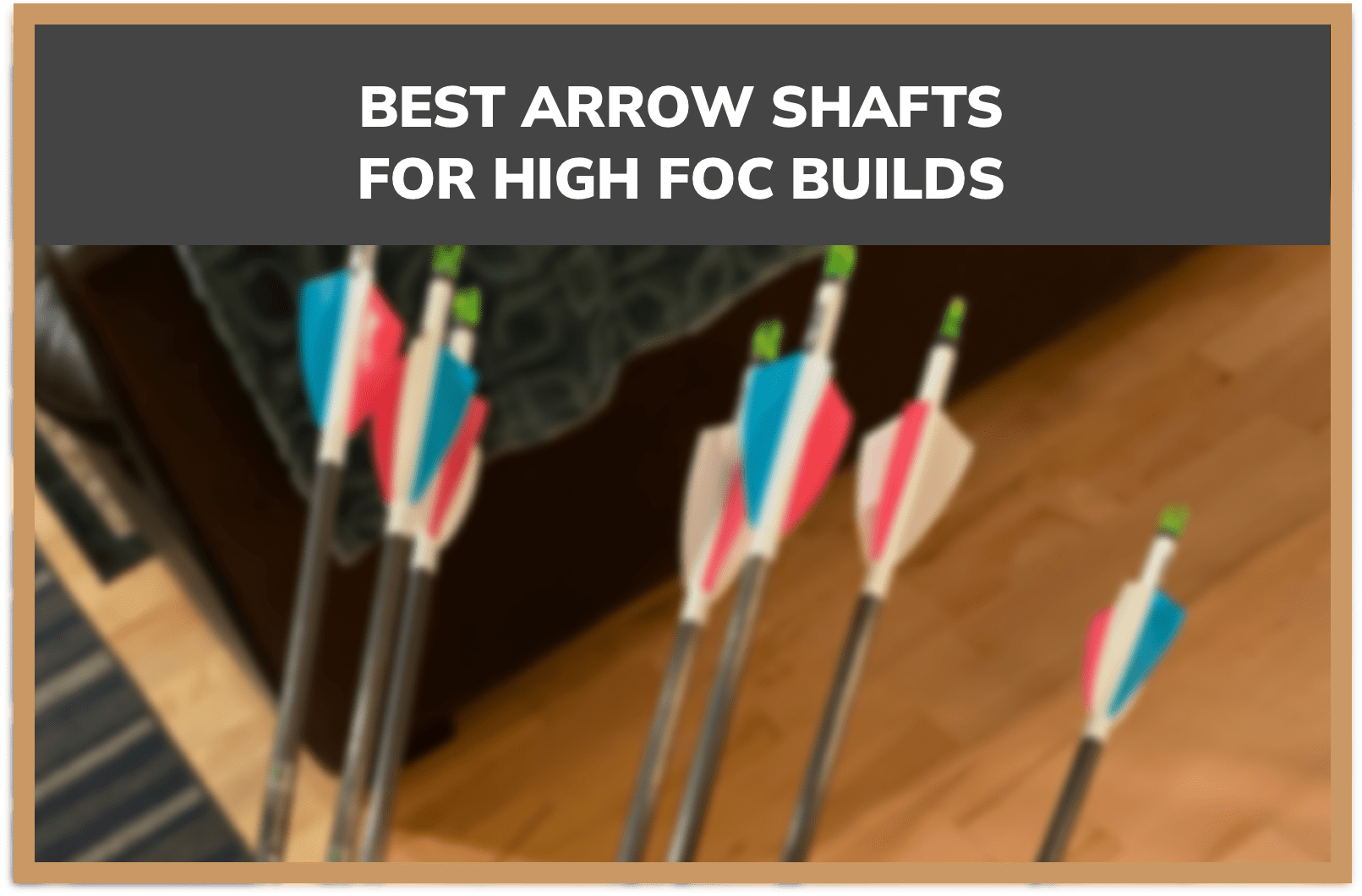# FOC Calculator

FOC Calculator2023-09-18T21:22:26+00:00Arrow FOC, or Front of Center, is an important factor in arrow flight and penetration. With our FOC calculator tools you can determine the FOC of your existing arrows, or you can calculate it for new arrows your are building. Start calculating below, or for definitions and details about FOC you can read more about how FOC works below.

## What is Arrow FOC (Front Of Center)?

In its most simple terms, arrow FOC represents the balance point of an arrow relative to its mid-point. More specifically, it measures how far the arrow's balance point is beyond the mid-point, and what percentage of the total arrow length that value is. To determine this, only the total arrow length and balance point of the arrow are needed. The arrow FOC formula, its input variables, and how to measure them are below.Total Arrow Length (L) - This is measured from the throat of the nock to the end of the arrow shaft (not including the point).

Balance Point of Arrow (BP) - This is measured from the throat of the nock to the balance point of the arrow. To find this for an existing arrow, take the arrow and balance it on an object, then measure the distance from that point to the nock throat.

### How do I calculate arrow FOC?

There are two methods if you are looking how to calculate arrow FOC. The first is by simple measurement of the input variables, which can be done on existing arrows. After you have measured the input variables as defined above, you can use our simple FOC calculator to calculate this value, or you can directly enter it into the arrow FOC formula.

For potential arrow builds, this calculation becomes significantly more difficult. While the arrow length is a simple calculation, the center of mass (balance point) must be determined theoretically based on the various attributes of the arrow. You can use our advanced FOC calculator to estimate the FOC for a variety of arrow configurations you are considering.

•### The best arrows for high FOC arrow buildsGo to Top